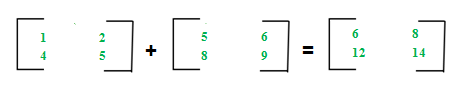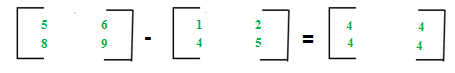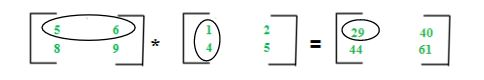# Different Operations on Matrices

• Difficulty Level : Easy
• Last Updated : 28 Jun, 2022

For introduction on matrices, you can refer the following article: Matrix Introduction
In this article, we will discuss various operations on matrices and their properties:

The addition of two matrices A m*n and Bm*n gives a matrix Cm*n. The elements of C are sum of corresponding elements in A and B which can be shown as:The algorithm for addition of matrices can be written as:

```for i in 1 to m
for j in 1 to n
cij = aij + bij```

## C++

 `// C++ Program for matrix addition` `#include ``using` `namespace` `std;` `int` `main()``{` `    ``int` `n = 2, m = 2;``    ``int` `a[n][m] = { { 2, 5 }, { 1, 7 } };``    ``int` `b[n][m] = { { 3, 7 }, { 2, 9 } };` `    ``int` `c[n][m];``    ``for` `(``int` `i = 0; i < n; i++)``        ``for` `(``int` `j = 0; j < n; j++) {``            ``c[i][j] = a[i][j] + b[i][j];``        ``}` `    ``for` `(``int` `i = 0; i < n; i++) {``        ``for` `(``int` `j = 0; j < n; j++)``            ``cout << c[i][j] << ``" "``;``        ``cout << endl;``    ``}``}`

## Java

 `// Java program for addition``// of two matrices``class` `GFG``{` `  ``// Driver code``  ``public` `static` `void` `main(String[] args)``  ``{``    ``int` `n = ``2``, m = ``2``;``    ``int` `a[][] = { { ``2``, ``5` `}, { ``1``, ``7` `} };` `    ``int` `b[][] = { { ``3``, ``7` `}, { ``2``, ``9` `} };` `    ``// To store result``    ``int` `c[][] = ``new` `int``[n][m];` `    ``for` `(``int` `i = ``0``; i < n; i++) {``      ``for` `(``int` `j = ``0``; j < m; j++)``        ``c[i][j] = a[i][j] + b[i][j];``    ``}` `    ``for` `(``int` `i = ``0``; i < n; i++) {``      ``for` `(``int` `j = ``0``; j < m; j++)``        ``System.out.print(c[i][j] + ``" "``);``      ``System.out.print(``"\n"``);``    ``}``  ``}``}` `// This code is contributed by Aarti_Rathi`

## Python3

 `# Python3 program for addition``# of two matrices` `N ``=` `4``# This function adds A[][]``# and B[][], and stores``# the result in C[][]`  `# driver code``a ``=` `[ [``2``, ``5``],``    ``[``1``, ``7``]]` `b``=` `[ [``3``, ``7``],``    ``[``2``, ``9``]]``    ` `N ``=` `2` `c``=``a[:][:] ``# To store result` `for` `i ``in` `range``(N):``    ``for` `j ``in` `range``(N):``        ``c[i][j] ``=` `a[i][j] ``+` `b[i][j]`  `for` `i ``in` `range``(N):``    ``for` `j ``in` `range``(N):``        ``print``(c[i][j], ``" "``, end``=``'')``    ``print``()``    ` `# This code is contributed by Aarti_Rathi`

## C#

 `// C# program to rotate a``// matrix by 90 degrees``using` `System;``class` `GFG {` `  ``// Driver Code``  ``static` `public` `void` `Main()``  ``{``    ``int` `N = 2;``    ``int` `M = 2;` `    ``// Test Case 1``    ``int``[,] a = { { 2, 5 }, { 1, 7 } };``    ``int``[,] b = { { 3, 7 }, { 2, 9 } };` `    ``int` `[,] c =``new` `int``[N,M];``    ``for` `(``int` `i = 0; i < N; i++)``      ``for` `(``int` `j = 0; j < M; j++) {``        ``c[i,j] = a[i,j] + b[i,j];``      ``}` `    ``for` `(``int` `i = 0; i < N; i++) {``      ``for` `(``int` `j = 0; j < M; j++)``        ``Console.Write(c[i,j] + ``" "``);``      ``Console.WriteLine();``    ``}``  ``}``}` `// This code is contributed by Aarti_Rathi`

## Javascript

 ``

Output:

```5 12
3 16 ```

Time Complexity: O(n * m)

Auxiliary Space: O(n * m)
Key points:

• Addition of matrices is commutative, which means A+B = B+A
• Addition of matrices is associative, which means A+(B+C) = (A+B)+C
• The order of matrices A, B, and A+B is always same
• If order of A and B is different, A+B can’t be computed
• The complexity of the addition operation is O(m*n) where m*n is order of matrices

Matrices Subtraction –
The subtraction of two matrices Am*n and Bm*n gives a matrix Cm*n. The elements of C are difference of corresponding elements in A and B which can be represented as:The algorithm for the subtraction of matrices can be written as:

```for i in 1 to m
for j in 1 to n
cij = aij-bij```

## C++

 `// C++ Program for matrix subtraction` `#include ``using` `namespace` `std;` `int` `main()``{` `    ``int` `n = 2, m = 2;``    ``int` `a[n][m] = { { 2, 5 }, { 1, 7 } };``    ``int` `b[n][m] = { { 3, 7 }, { 2, 9 } };` `    ``int` `c[n][m];``    ``for` `(``int` `i = 0; i < n; i++)``        ``for` `(``int` `j = 0; j < n; j++) {``            ``c[i][j] = a[i][j] - b[i][j];``        ``}` `    ``for` `(``int` `i = 0; i < n; i++) {``        ``for` `(``int` `j = 0; j < n; j++)``            ``cout << c[i][j] << ``" "``;``        ``cout << endl;``    ``}``}`

## Java

 `public` `class` `GFG {``    ``// Java Program for matrix subtraction` `    ``public` `static` `void` `main(String[] args)``    ``{` `        ``int` `n = ``2``;``        ``int` `m = ``2``;``        ``int``[][] a = { { ``2``, ``5` `}, { ``1``, ``7` `} };``        ``int``[][] b = { { ``3``, ``7` `}, { ``2``, ``9` `} };` `        ``int``[][] c = ``new` `int``[n][m];``        ``for` `(``int` `i = ``0``; i < n; i++) {``            ``for` `(``int` `j = ``0``; j < n; j++) {``                ``c[i][j] = a[i][j] - b[i][j];``            ``}``        ``}` `        ``for` `(``int` `i = ``0``; i < n; i++) {``            ``for` `(``int` `j = ``0``; j < n; j++) {``                ``System.out.print(c[i][j]);``                ``System.out.print(``" "``);``            ``}``            ``System.out.print(``"\n"``);``        ``}``    ``}``}` `// This code is contributed by Aarti_Rathi`

## Python3

 `# Python3 program for addition``# of two matrices``N ``=` `4` `# This function adds A[][]``# and B[][], and stores``# the result in C[][]` `# driver code``a ``=` `[[``2``, ``5``],``     ``[``1``, ``7``]]` `b ``=` `[[``3``, ``7``],``     ``[``2``, ``9``]]` `N ``=` `2` `c ``=` `a[:][:]  ``# To store result` `for` `i ``in` `range``(N):``    ``for` `j ``in` `range``(N):``        ``c[i][j] ``=` `a[i][j] ``-` `b[i][j]`  `for` `i ``in` `range``(N):``    ``for` `j ``in` `range``(N):``        ``print``(c[i][j], ``" "``, end``=``'')``    ``print``()` `# This code is contributed by Aarti_Rathi`

## C#

 `// C# program to rotate a``// matrix by 90 degrees``using` `System;`` ` `class` `GFG {`` ` `     ``// Driver Code``    ``static` `public` `void` `Main()``    ``{``        ``int` `N = 2;``        ``int` `M = 2;``       ` `        ``// Test Case 1``        ``int``[,] a = { { 2, 5 }, { 1, 7 } };``        ``int``[,] b = { { 3, 7 }, { 2, 9 } };`` ` `        ``int` `[,] c =``new` `int``[N,M];``        ``for` `(``int` `i = 0; i < N; i++)``            ``for` `(``int` `j = 0; j < M; j++) {``                ``c[i,j] = a[i,j] - b[i,j];``            ``}``     ` `        ``for` `(``int` `i = 0; i < N; i++) {``            ``for` `(``int` `j = 0; j < M; j++)``                ``Console.Write(c[i,j] + ``" "``);``            ``Console.WriteLine();``        ``}``    ``}``}`` ` `// This code is contributed by Aarti_Rathi`

## Javascript

 ``

Output:

```-1 -2
-1 -2 ```

Key points:

• Subtraction of matrices is non-commutative which means A-B ≠ B-A
• Subtraction of matrices is non-associative which means A-(B-C) ≠ (A-B)-C
• The order of matrices A, B and A-B is always same
• If order of A and B is different, A-B can’t be computed
• The complexity of subtraction operation is O(m*n) where m*n is order of matrices

Matrices Multiplication –
The multiplication of two matrices Am*n and Bn*p gives a matrix Cm*p. It means number of columns in A must be equal to number of rows in B to calculate C=A*B. To calculate element c11, multiply elements of 1st row of A with 1st column of B and add them (5*1+6*4) which can be shown as:The algorithm for multiplication of matrices A with order m*n and B with order n*p can be written as:

```for i in 1 to m
for j in 1 to p
cij = 0
for k in 1 to n
cij += aik*bkj```

## C++

 `// C++ Program for matrix Multiplication` `#include ``using` `namespace` `std;` `int` `main()``{` `    ``int` `n = 2, m = 2;``    ``int` `a[n][m] = { { 2, 5 }, { 1, 7 } };``    ``int` `b[n][m] = { { 3, 7 }, { 2, 9 } };` `    ``int` `c[n][m];``    ``int` `i, j, k;``    ``for` `(i = 0; i < n; i++)``    ``{``        ``for` `(j = 0; j < n; j++)``        ``{``            ``c[i][j] = 0;``            ``for` `(k = 0; k < n; k++)``                ``c[i][j] += a[i][k] * b[k][j];``        ``}``    ``}` `    ``for` `(``int` `i = 0; i < n; i++)``    ``{``        ``for` `(``int` `j = 0; j < n; j++)``            ``cout << c[i][j] << ``" "``;``        ``cout << endl;``    ``}``}`

## C#

 `// C# program to rotate a``// matrix by 90 degrees``using` `System;` `class` `GFG {` `  ``// Driver Code``  ``static` `public` `void` `Main()``  ``{``    ``int` `N = 2;``    ``int` `M = 2;` `    ``// Test Case 1``    ``int``[,] a = { { 2, 5 }, { 1, 7 } };``    ``int``[,] b = { { 3, 7 }, { 2, 9 } };` `    ``int` `[,] c =``new` `int``[N,M];` `    ``for` `(``int` `i = 0; i < N; i++)``    ``{``      ``for` `(``int` `j = 0; j < M; j++) {``        ``c[i,j]=0;``        ``for``(``int` `k=0;k

## Javascript

 ``

Output:

```16 59
17 70```

Key points:

• Multiplication of matrices is non-commutative which means A*B ≠ B*A
• Multiplication of matrices is associative which means A*(B*C) = (A*B)*C
• For computing A*B, the number of columns in A must be equal to number of rows in B
• Existence of A*B does not imply existence of B*A
• The complexity of multiplication operation (A*B) is O(m*n*p) where m*n and n*p are order of A and B respectively
• The order of matrix C computed as A*B is m*p where m*n and n*p are order of A and B respectively

My Personal Notes arrow_drop_up